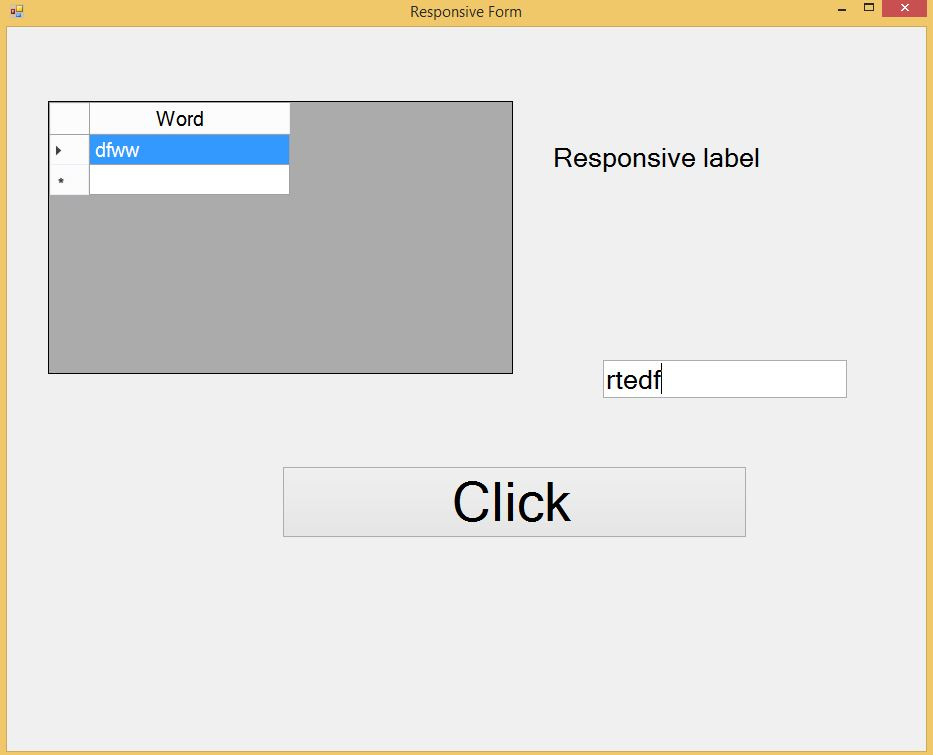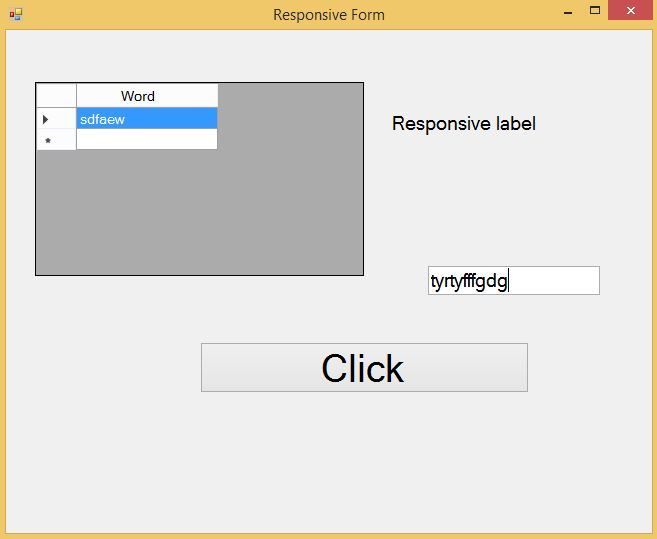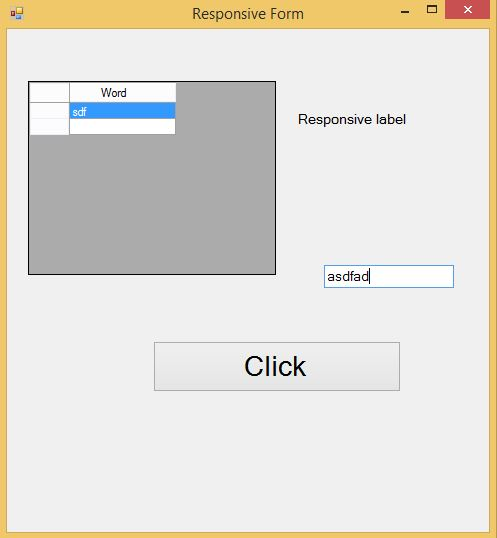## 代码

### The Responsive Class – Responsive.cs

``````float WIDTH_AT_DESIGN_TIME = (float)Convert.ToDouble
(ConfigurationManager.AppSettings["DESIGN_TIME_SCREEN_WIDTH"]);
float HEIGHT_AT_DESIGN_TIME = (float)Convert.ToDouble
(ConfigurationManager.AppSettings["DESIGN_TIME_SCREEN_HEIGHT"]);
Rectangle Resolution;
float WidthMultiplicationFactor;
float HeightMultiplicationFactor;
``````

``````<add key ="DESIGN_TIME_SCREEN_WIDTH" value="1920"/>
``````

``````public Responsive(Rectangle ResolutionParam)
{
Resolution = ResolutionParam;
}

public void SetMultiplicationFactor()
{
WidthMultiplicationFactor = Resolution.Width / WIDTH_AT_DESIGN_TIME;
HeightMultiplicationFactor = Resolution.Height / HEIGHT_AT_DESIGN_TIME;
}
``````

``````WidthMultiplicationFactor = 1024/1920 = 0.533
HeightMultiplicationFactor = 768/1080 = 0.711
``````

``````public int GetMetrics(int ComponentValue)
{
return (int)(Math.Floor(ComponentValue * WidthMultiplicationFactor));
}

public int GetMetrics(int ComponentValue, string Direction)
{
if (Direction.Equals("Width") || Direction.Equals("Left"))
return (int)(Math.Floor(ComponentValue * WidthMultiplicationFactor));
else if (Direction.Equals("Height") || Direction.Equals("Top"))
return (int)(Math.Floor(ComponentValue * HeightMultiplicationFactor));
return 1;
}
``````

``````Width = 465 * 0.533 = 248
Height = 72 * 0.711= 51
Left = 366 * 0.533= 195
Top = 41 * 0.711= 29
Font-size = 40 * 0.533 = 21
``````

### The Responsive Class – Responsive.cs

``````float WIDTH_AT_DESIGN_TIME = (float)Convert.ToDouble
(ConfigurationManager.AppSettings["DESIGN_TIME_SCREEN_WIDTH"]);
float HEIGHT_AT_DESIGN_TIME = (float)Convert.ToDouble
(ConfigurationManager.AppSettings["DESIGN_TIME_SCREEN_HEIGHT"]);
Rectangle Resolution;
float WidthMultiplicationFactor;
float HeightMultiplicationFactor;
``````

``````<add key ="DESIGN_TIME_SCREEN_WIDTH" value="1920"/>
``````

``````public Responsive(Rectangle ResolutionParam)
{
Resolution = ResolutionParam;
}

public void SetMultiplicationFactor()
{
WidthMultiplicationFactor = Resolution.Width / WIDTH_AT_DESIGN_TIME;
HeightMultiplicationFactor = Resolution.Height / HEIGHT_AT_DESIGN_TIME;
}
``````

``````WidthMultiplicationFactor = 1024/1920 = 0.533
HeightMultiplicationFactor = 768/1080 = 0.711
``````

``````public int GetMetrics(int ComponentValue)
{
return (int)(Math.Floor(ComponentValue * WidthMultiplicationFactor));
}

public int GetMetrics(int ComponentValue, string Direction)
{
if (Direction.Equals("Width") || Direction.Equals("Left"))
return (int)(Math.Floor(ComponentValue * WidthMultiplicationFactor));
else if (Direction.Equals("Height") || Direction.Equals("Top"))
return (int)(Math.Floor(ComponentValue * HeightMultiplicationFactor));
return 1;
}
``````

``````Width = 465 * 0.533 = 248
Height = 72 * 0.711= 51
Left = 366 * 0.533= 195
Top = 41 * 0.711= 29
Font-size = 40 * 0.533 = 21
``````

### 使用 Responsive Class

``````Responsive ResponsiveObj;
ResponsiveObj = new Responsive(Screen.PrimaryScreen.Bounds);
ResponsiveObj.SetMultiplicationFactor();
``````

``````private void ResponsiveForm_Load(object sender, EventArgs e)
{
Width = ResponsiveObj.GetMetrics(Width, "Width");           // Form width and height set up.
Height = ResponsiveObj.GetMetrics(Height, "Height");
Left = Screen.GetBounds(this).Width / 2 - Width / 2;        // Form centering.
Top = Screen.GetBounds(this).Height / 2 - Height / 2 - 30;  // 30 is a calibration factor.

foreach (Control Ctl in this.Controls)
{
Ctl.Font = new Font(FontFamily.GenericSansSerif,
ResponsiveObj.GetMetrics((int)Ctl.Font.Size), FontStyle.Regular);
Ctl.Width = ResponsiveObj.GetMetrics(Ctl.Width, "Width");
Ctl.Height = ResponsiveObj.GetMetrics(Ctl.Height, "Height");
Ctl.Top = ResponsiveObj.GetMetrics(Ctl.Top, "Top");
Ctl.Left = ResponsiveObj.GetMetrics(Ctl.Left, "Left");
}
}
``````

### 使用 Responsive Class

``````Responsive ResponsiveObj;
ResponsiveObj = new Responsive(Screen.PrimaryScreen.Bounds);
ResponsiveObj.SetMultiplicationFactor();
``````

``````private void ResponsiveForm_Load(object sender, EventArgs e)
{
Width = ResponsiveObj.GetMetrics(Width, "Width");           // Form width and height set up.
Height = ResponsiveObj.GetMetrics(Height, "Height");
Left = Screen.GetBounds(this).Width / 2 - Width / 2;        // Form centering.
Top = Screen.GetBounds(this).Height / 2 - Height / 2 - 30;  // 30 is a calibration factor.

foreach (Control Ctl in this.Controls)
{
Ctl.Font = new Font(FontFamily.GenericSansSerif,
ResponsiveObj.GetMetrics((int)Ctl.Font.Size), FontStyle.Regular);
Ctl.Width = ResponsiveObj.GetMetrics(Ctl.Width, "Width");
Ctl.Height = ResponsiveObj.GetMetrics(Ctl.Height, "Height");
Ctl.Top = ResponsiveObj.GetMetrics(Ctl.Top, "Top");
Ctl.Left = ResponsiveObj.GetMetrics(Ctl.Left, "Left");
}
}
``````

### 示例

gird，一个label，一个textbox和一个button。### 示例

gird，一个label，一个textbox和一个button。## 代码调整

``````private void ResponsiveForm_Load(object sender, EventArgs e)
{
Width = ResponsiveObj.GetMetrics(Width, "Width");           // Form width and height set up.
Height = ResponsiveObj.GetMetrics(Height, "Height");
Left = Screen.GetBounds(this).Width / 2 - Width / 2;        // Form centering.
Top = Screen.GetBounds(this).Height / 2 - Height / 2 - 30;  // 30 is a calibration factor.

foreach (Control Ctl in this.Controls)
{
if (Ctl is PictureBox)
{
Ctl.Width = ResponsiveObj.GetMetrics(Ctl.Width, "Width");
Ctl.Height = ResponsiveObj.GetMetrics(Ctl.Height, "Height");
Ctl.Top = ResponsiveObj.GetMetrics(Ctl.Top, "Top");
Ctl.Left = ResponsiveObj.GetMetrics(Ctl.Left, "Left");
}
else
{
Ctl.Font = new Font(FontFamily.GenericSansSerif,
ResponsiveObj.GetMetrics((int)Ctl.Font.Size), FontStyle.Regular);
Ctl.Width = ResponsiveObj.GetMetrics(Ctl.Width, "Width");
Ctl.Height = ResponsiveObj.GetMetrics(Ctl.Height, "Height");
Ctl.Top = ResponsiveObj.GetMetrics(Ctl.Top, "Top");
Ctl.Left = ResponsiveObj.GetMetrics(Ctl.Left, "Left");
}
}
}
``````

## 代码调整

``````private void ResponsiveForm_Load(object sender, EventArgs e)
{
Width = ResponsiveObj.GetMetrics(Width, "Width");           // Form width and height set up.
Height = ResponsiveObj.GetMetrics(Height, "Height");
Left = Screen.GetBounds(this).Width / 2 - Width / 2;        // Form centering.
Top = Screen.GetBounds(this).Height / 2 - Height / 2 - 30;  // 30 is a calibration factor.

foreach (Control Ctl in this.Controls)
{
if (Ctl is PictureBox)
{
Ctl.Width = ResponsiveObj.GetMetrics(Ctl.Width, "Width");
Ctl.Height = ResponsiveObj.GetMetrics(Ctl.Height, "Height");
Ctl.Top = ResponsiveObj.GetMetrics(Ctl.Top, "Top");
Ctl.Left = ResponsiveObj.GetMetrics(Ctl.Left, "Left");
}
else
{
Ctl.Font = new Font(FontFamily.GenericSansSerif,
ResponsiveObj.GetMetrics((int)Ctl.Font.Size), FontStyle.Regular);
Ctl.Width = ResponsiveObj.GetMetrics(Ctl.Width, "Width");
Ctl.Height = ResponsiveObj.GetMetrics(Ctl.Height, "Height");
Ctl.Top = ResponsiveObj.GetMetrics(Ctl.Top, "Top");
Ctl.Left = ResponsiveObj.GetMetrics(Ctl.Left, "Left");
}
}
}
``````

So easy！

So easy！